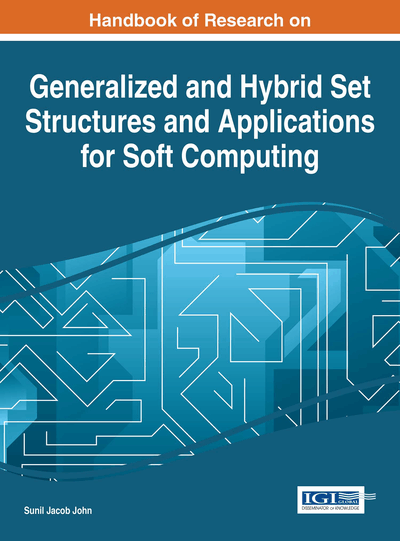Fuzzy Multi-Criteria Assignment Problems Using Two-Dimensional Fuzzy Soft Set Theory

Shyamal Kumar Mondal (Vidyasagar University, India)
DOI: 10.4018/978-1-4666-9798-0.ch010

Abstract

In this chapter, author has introduced a new concept of two-dimensional fuzzy soft sets together with various operations, properties and theorems on them. Then, an algorithm named 2-DFS has been developed for solving fuzzy multi-criteria assignment problems with multiple decision makers. The performance of this newly proposed method is verified by the popular Hungarian Method in case of solving fuzzy assignment problems with single criterion and single decision maker. By Hungarian Method, one may only solve fuzzy assignment problems with single criterion and single decision maker, in the contrary the advantage of our 2-DFS Algorithm is that by it, any fuzzy assignment problem with any number of criteria and any number of decision makers can be solved effectively. At last,2- DFS Algorithm is applied for solving fuzzy multi-criteria assignment problems in medical science to evaluate the effectiveness of different modalities of treatment of a disease.
Chapter Preview
Top

1 Introduction

Most of the recent mathematical methods meant for formal modeling, reasoning and computing are crisp, accurate and deterministic in nature. But in ground reality, crisp data is not always the part and parcel of the problems encountered in different fields like economics, engineering, social science, medical science, environment etc. As a consequence, various theories viz. theory of probability, theory of fuzzy sets (Zadeh, 1965), theory of Intuitionistic fuzzy sets (Atanassov, 1986), theory of vague sets (Gau & Buehrer, 1993), theory of interval mathematics (Gorzalczany, 1987), theory of rough sets (Pawlak, 1982) have been evolved in process.

But, difficulties present in all these theories have been shown by Molodtsov (1999). The cause of these problems is possibly related to the inadequacy of the parameterization tool of the theories. As a result, Molodtsov (1999) proposed the concept of soft theory as a new mathematical tool for solving the uncertainties which is free from the above difficulties. Soft set is a parameterized general mathematical tool which deals with a collection of approximate descriptions of objects. Each approximate description has two parts, a predicate and an approximate value set. In classical mathematics, a mathematical model of an object is constructed and it defines the notion of exact solution of this model. Usually, the mathematical model is too complicated and the exact solution is not easily obtained. So, the notion of approximate solution is introduced and the solution is calculated. In the soft set theory, we have the opposite approach to this problem. The initial description of the object has an approximate nature, and we do not need to introduce the notion of exact solution. The absence of any restrictions on the approximate description in soft set theory makes this theory very convenient and easily applicable in practice. Soft sets could be regarded as neighborhood systems, and they are a special case of context-dependent fuzzy sets. In soft set theory, the problem of setting the membership function, among other related problems, simply does not arise. This makes the theory very convenient and easy to apply in practice. Maji et al. (2002, 2003) have done further research on soft set theory.

In the literature of fuzzy sets (Zimmerman (1996), Zadeh (1965)), the word fuzzy often stands for the word vague. Some comments on the links between vagueness and fuzziness are useful. In common use, there is a property of objects called “fuzziness”. From the Oxford English Dictionary we read that “fuzzy” means either not firm or sound in substance, or fringed into loose fibers. Fuzzy means also covered by fuzz, i.e., with loose volatile matter. Alike any other characteristics, “fuzzy” can be used to form a predicate of the form: “something is fuzzy”. For example “a bear is fuzzy”. It may sound strange to say that “bald is fuzzy”, or that “young is fuzzy”. Words (adjectives in this case) bald and young are vague (but not fuzzy in the material sense) because their meanings are not fixed by sharp boundaries. Similarly, objects are not vague. However, the word “fuzzy” is applied to words, especially predicates, and is supposed to refer to the gradual nature of some of these words, which causes them to appear as vague.

The presence of vagueness demanded Fuzzy Soft Set (Basu et al., (2012)) to come into picture. Maji & Roy (2001, 2009, 2007) have presented an algorithm for solving fuzzy soft set based decision making problems. In 2009, Kharal & Ahamad have defined the notion of a mapping on classes of fuzzy soft sets. Li (2010) has discussed the relation between soft lattices and fuzzy soft sets. Then, Feng et al. (2010) have proposed an adjustable approach to FSS based decision making by means of level soft sets. Deli et al. (2015) have proposed Intuitionistic fuzzy parameterized soft set theory and its decision making.

Complete Chapter List

Search this Book:
Reset